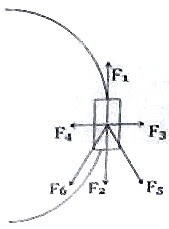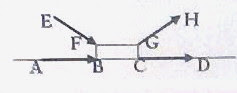## Pages

`“Life is like riding a bicycle.  To keep your balance you must keep moving.”–Albert Einstein`

## Sunday, October 12, 2008

### AP Physics – Additional Questions (MCQ) on Newton’s Laws

As promised in the last post, I give below a few more multiple choice questions (with solution) on Newton’s Laws:

(1) The string of a simple pendulum inside a car (fig.) makes an angle of 30º with the vertical when the car accelerates along horizontal road AB. Pick out the correct option from the following:(a) The car is moving from A to B with acceleration approximately 5 ms–2

(b) The car is moving from B to A with acceleration approximately 5 ms–2

(c) The car is moving from B to A with acceleration approximately 10 ms–2

(d) The car is moving from A to B with acceleration approximately 7 ms–2

(e) The car is moving from B to A with acceleration approximately 7 ms–2The string of the pendulum makes an angle with the vertical because of the inertial force on the bob of the pendulum. Since the bob is deflected towards B, the car is moving from B to A. The inertial force on the bob is ma where m is the mass of the bob and a is the acceleration of the car. The bob is in equilibrium under the action of three forces: (i) weight mg of the bob, (ii) inertial force ma on the bob and (iii) tension T in the string. These can be represented by the triangle of forces ABC so that AB, BC and CA represent the forces mg, ma and T respectively. Therefore, we have

tan 30º = ma/mg from which a = 10/√2 ms–2, on substituting g = 10 ms–2 approximately. This works out to nearly 7 ms–2.

The correct option is (e).

(2) In the adjoining figure F1 represents the viscous drag force (due to air) on a car negotiating a circular curve on level ground. Which one among the other forces shown in the figure most appropriately represents the resultant horizontal frictional force acting on the tyres of the car?(a) F1

(b) F2

(c) F3

(d) F4

(e) F5

The above question is meant for gauging your understanding of frictional force which moves a car along a road. Since the viscous drag force (due to air) on the car is represented by F1, the car is moving opposite to F1. At the poinf of contact on the road, the tyre is moving in the direction of F1 and the friction which moves the car forward is opposite to F1 and is to be represented by F2. The car is prevented from skidding by the component of friction acting radially inwards and is represented by F4. The resultant of F2 and F4 is represented by F6 which is the net horizontal frictional force acting on the tyres of the car.

(3) A boy slides a heavy box (fig.) along a horizontal rough surface by equal distances by applying a force in four different ways. Case (i): He pushes the box horizontally, applying the force along AB. Case (ii): He pulls the box horizontally, applying the force along CD. Case (iii): He pushes the box by applying the force along EF. Case (iv): He pulls the box by applying the force along GH. In which case/s he will have to do the least amount of work?(a) Case (ii)

(b) Case (iii)

(c) Case (iv)

(d) Cases (ii) and (iv)

(e) Cases (i), (ii) and (iv)

The boy need not do any work against gravity since he slides the box horizontally. Work has to be done against frictional force. Recall that the frictional force is μN where μ is the coefficient of friction and N is the normal force.Frictional force is least in case (iv) since the normal force is least and is equal to (mg F sinθ). In cases (i) and (ii) the normal force is mg. In case (iii) the normal force is (mg + F sinθ).

The boy will have to do the least amount of work in case (iv).

[Is it not easier to pull a lawn mower than to push it?]

(4) Two boys pull the ends of a light inextensible cord so that a tension of 50 N is developed in the cord. The forces exerted by them are

(a) 50 N and 50 N

(b) 100 N and 100 N

(c) 25 N and 25 N

(d) 30 N and 20 N

(e) given by options (c) and (d)

If one end of the cord is tied to a hook on the wall and one of the boys pulls the other end with a force of 50 N, what will be the tension in the cord? You will give the answer as 50 N with out any doubt. The second boy just functions as the hook and the answer to our problem is 50 N and 50 N [Option (a)].

(5) A block of wood is placed on a rough inclined plane whose inclination is gradually increased from zero. The frictional force between the block and the plane